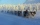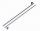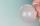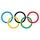# This morning

This morning it was 80 degrees F outside the temperature increased 12 degrees F. What is the temperature now?

Result

t =  92 °F

#### Solution:Leave us a comment of example and its solution (i.e. if it is still somewhat unclear...):

Showing 0 comments:Be the first to comment!## Next similar examples:

1. Thermometerthermometer showed -12 degrees Celsius at the morning then the temperature rises by 4 degrees and later again increased by 2 degrees at the evening has fallen by 5 degrees and then fall 3 degrees. What end temperature does the thermometer show?
2. Adding mixed numbersAdd this two mixed numbers: 1 5/6 + 2 2/11=
3. NoontimeIn the morning temperature was 110°F. By noontime it has gone up by 15°F. What was the noon temperature. (Give your answer in °F)
4. Outside temperatureThe temperature outside was 57 degree Fahrenheit. During the next few hours it decreased by 18 degrees and then increased by 23 degrees. Find new temperature.
5. Temperature 2Sunday's high temperature was 3 degrees higher than Saturday's. On Monday, the temperature fell 5 degrees, then rose 7 degrees on Tuesday and 4 more on Wednesday. Then it fell 17 degrees to a record low of 31 on Thursday. What was the temperature on Saturd
6. The temperartureThe temperarture is 0 degrees. It drops 5 degrees after 1 hour and then drops 3 degrees more the next hour. What is the temperature after 6 hours?
7. Temperature addingAt a weather centre, the temperature at midnight was -2 degree Celsius and by noon it had raised 4 degree Celsius. What is the new temperature?
8. Change in temperatureStarting temperature is 21°C, the highest temperature is 32°C. What is the change in temperature?
9. Roman numerals +Add up the number writtens in Roman numerals. Write the results as a decimal number.
10. Addition of Roman numbersAdded together and write as decimal number: LXVII + MLXIV
11. Roman numerals 2+Add up the number writtens in Roman numerals. Write the results as a roman numbers.
12. Addition of mixed numeralsAdd two mixed fractions: 2 4/6 + 1 3/6
13. Roses and tulipsAt the florist are 50 tulips and 5 times less roses. How many flowers are in flower shop?
14. CollectionMajka gave from her collection of calendars Hanke 15 calendars, Julke 6 calendars and Petke 10 calendars. Still remains 77 calendars. How many calendars had Majka in her collection at the beginning?
15. PlaneOn how many parts divide plane 6 parallels?
16. Ten pupils10 pupils went to the store. 6 pupils bought lollipops and 9 pupils bought chewing gum. How many pupils have bought both lollipops and chewing gums (if everyone bought something)?
17. CirclesFor the circle c1(S1; r1=146 cm) and c2(S2; r2 = 144 cm) is distance of centers |S1S2| = 295 cm. Determine the distance between the circles.